﻿ 5. Detailed description of the Actions > 5.5. Standard > 5.5.5. Aggregate (Group by) (High-Speed action) > 5.5.5.3. Using the “out-of-memory mode” inside a N-Way Multithreaded Section

# 5.5.5.3. Using the “out-of-memory mode” inside a N-Way Multithreaded Section

Let’s assume that we want to multithread/parallelize this simple Anatella Transformation Graph: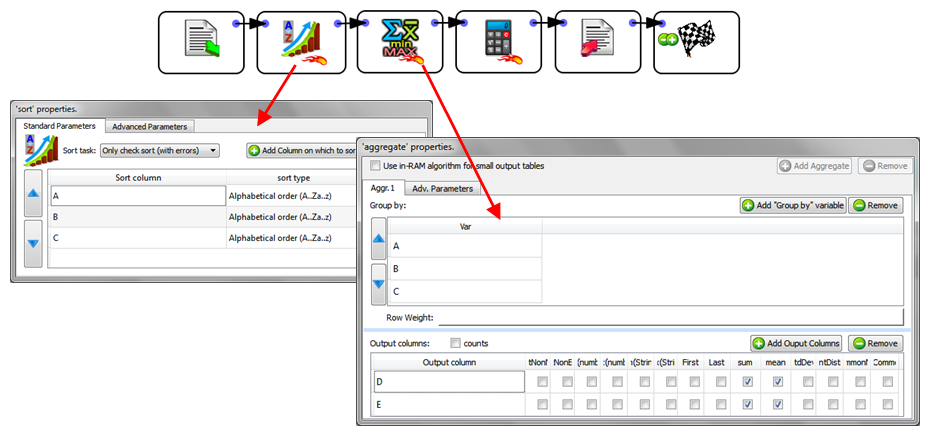When the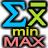Aggregate Action starts, it looks at the meta-data of the input table to check if it’s properly sorted on the columns A, B & C. …And since that’s the case (because of the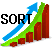Sort Action running in “Check sort with error” mode), it can proceed computing the aggregations.

The above graph is equivalent to the SQL command:

“SELECT sum(D) as D_sum,

sum(E) as E_sum,

mean(D) as D_mean,

mean(E) as E_mean,

FROM   table

GROUP BY   A,B,C

…followed by some small computation based on A, B, C, D, D_sum, E_sum, D_mean, E_mean.

Let’s now include theAggregate Action, inside a N-Way Multithread Section:By default, the above graph won’t run because the meta-data of the input table of theAggregate Action says that the input table is not sorted (by default, any sort-meta-data is lost at the start of the N-Way Multithread Section). To “keep” the sort-meta-data inside the interior of the N-Way Multithread section, we must set the partitioning parameter of the second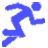Multithread Action to “A”: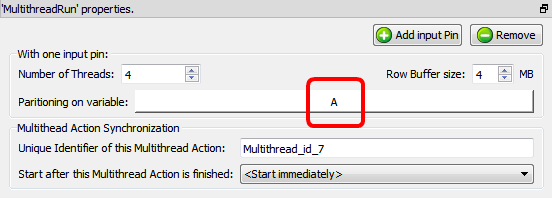This is a special case: When the partitioning parameter is equal to the most significant column of the sort-meta-data, then the sort meta-data is kept inside the interior of the N-Way Multithread section. (So that theAggregate Action works, once again, properly)

Let’s now assume that the text file that is used as source data for the Anatella graph is sorted on the column A only (and not on the columns A, B & C, as previously). We’ll thus have:

In the general case, the output table of the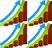Partitioned Sort Action is not sorted (i.e. it does not contain any sort-meta-data at all). In the above example, we are in a special case: The partitioning variable of thePartitioned Sort Action is equal to the most-significant sort-variable of the input table. In this special case, the sort-meta-data of the output table is not empty (see section 5.5.2). In the above example, the sort-meta-data is automatically set to: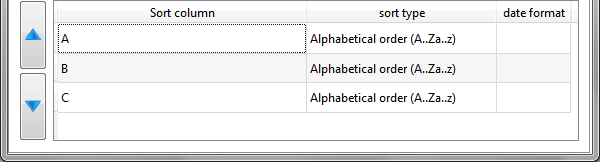So that theAggregate Action works, once again, properly!)

The above Anatella graph is very efficient because:

a)It computes aggregations using many CPUs (because theAggregate Action is inside a N-Way Multithread section).

b)The aggregations are computed using the “out-of-memory” mode, meaning that we can handle output tables of unlimited size.

c)When using the “out-of-memory” mode to compute aggregation, the input table must be sorted on all the “group by” variables. In the above example, the input table was only partially sorted on one of the “group by” variable (i.e. it was sorted only on the column A, but not on the columns B and C). Although the source table was not properly sorted, we were nevertheless able to compute the aggregations, thanks to thePartitioned Sort Action that “extended” the sort-meta-data (to include the columns B and C) so that theAggregate Action still works properly.

d)We were able to run on many CPUs thePartitioned Sort Action (because it’s inside a N-Way Multithread section). Usually, sorting is a very slow operation and thus it’s very nice to be able to easily use many CPU’s to sort the data because it reduces considerably the computation time.

e)It’s using a very small amount of RAM memory (there are noSort Action and theAggregate Action are running in “out-of-memory” mode).